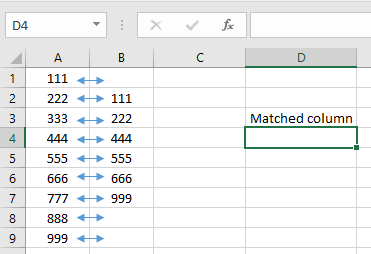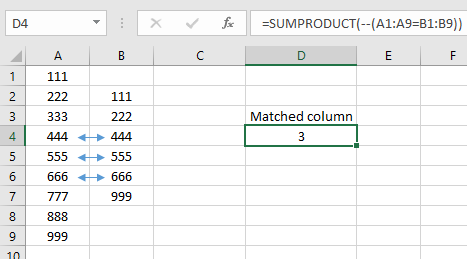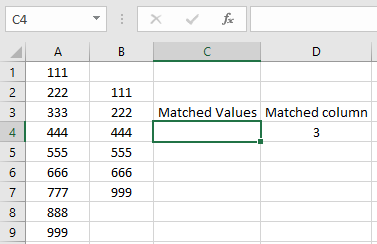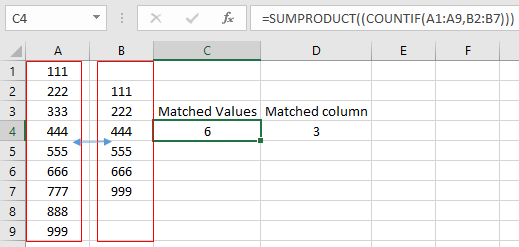# How to Count total matches in two ranges in Excel

In this article, we will learn about how to count total matches in Excel. SUMPRODUCT function gets the sum of all the product values.-- is used to interpret the results to the 0s and 1s Syntax:

=SUMPRODUCT(--(range1=range2))

Length of range1 = Length of range2 or otherwise it will give #NA error
Example:
Let’s understand this function using it in an example.Here we have 2 list of values and we need to check how many values are matched column wise. Use the formula:

=SUMPRODUCT(--(A1:A9=B1:B9))

Explanation: A1:A9=B1:B9 : The function checks corresponding value and returns TRUE or FALSE based on value is matched or not -- : This converts all TRUE to 1s and all FALSE to 0s. Then the sumproduct takes the sum of all values after conversion.As you can see the formula returns 3. That means there are 3 values matched. Now we wish to Count all the matches from range1 to range2. For this we will use a combination of SUMPRODUCT & COUNTIF function. COUNTIF function returns the count of values where conditions meet. Syntax:

=COUNTIF (range, criteria)Here we take the same table and count the matched values in both ranges. Now use the formula:

=SUMPRODUCT((COUNTIF(A1:A9,B2:B7)))

Length of range1 not necessary to be equal to Length of range2 Explanation: Range1 checks values equivalency with range 2 and gives a list of 1s and 0s. SUMPRODUCT function gets the sum of all.As you can see 6 values matched from the 2 ranges. Hope you understood how to and referring cell in Excel. Explore more articles on Excel cell reference function here. Please feel free to state your query or feedback for the above article.

Related Articles:

How to Count Cells that contain specific text in Excel

How to use the COUNT Function in Excel

How to use the COUNTA Function in Excel

Popular Articles:

How to use the VLOOKUP Function in Excel

How to use the COUNTIF function in Excel

How to use the SUMIF Function in Excel

Terms and Conditions of use

The applications/code on this site are distributed as is and without warranties or liability. In no event shall the owner of the copyrights, or the authors of the applications/code be liable for any loss of profit, any problems or any damage resulting from the use or evaluation of the applications/code.